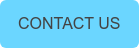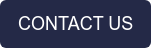#### Understanding the Viscosity of Liquids

Matter is broken down into three groups: solids, liquids, and gases. Each of these types of matter are different in the way that they move, stick together, and break apart. Liquids, for instance, are unique in their ability to move somewhat freely in aggregated groups of molecules. Likewise, solids are unique for the ways that they break apart from their rigid structures into pieces, and gases for the way that the molecules move independently of each other. Understanding the traits of matter, especially the ways that they flow and break, is very important for a wide variety of professions, including those in materials production.

The field of study surrounding certain traits of matter is called rheology.

# Defining Rheology

The word rheology comes from the Greek word "rheos," translated to English as "stream," and it might remind some of the Spanish word "rio." This is important to understand the origin of the word because rheology is the study of the flow (like a stream or a river) and the subsequent deformation of matter as a result of flow. The rheological characteristics of materials directly affect the way that they should be handled and processed. Specifically, the rheological properties determine:

• How the material should be mixed
• What tools should be used to disperse the material
• The way that coatings settle,
• The material's shear rate, or the rate that the material can be deformed
• How the material flows into spaces.

These rheological traits are affected by certain inherent qualities of the material, namely:

• Resin, which affects the viscosity and the surface wetting of the material
• Formulation of the material with its additives
• Temperature, which directly affects the viscosity of the material
• Shear, or the way that a material reacts to force.

Understanding all of these traits is fundamental for working with liquids at any level of production. The machinery that is used to disperse and mix liquid materials is related directly to the kind of material and its rheological properties.

### How is Rheology Classified?

Rheology has developed two classes of liquids: Newtonian and Non-Newtonian fluids.

### Newtonian Fluids

Newtonian fluids are those which follow Newton's hypothesis, and they are considered to be perfectly viscous. This is because the ratio between the shear rate and shear stress are constant, or in other words, the viscosity of the liquid remains constant at all possible shear rates for a given temperature. Pure water, oils, and organic solvents are all examples of Newtonian liquids. Because of their purity and the lack of dispersion, Newtonian fluids are much easier to measure. Unfortunately, however, they are not very common.

### Non-Newtonian Fluids

Most liquids are non-Newtonian fluids, meaning they do not have a constant ratio between their shear rate and shear stress. These fluids can be unpredictable in how their shear stress changes according to the shear rate: as the shear rate increases, the shear stress can either increase or decrease, depending on the fluid's own characteristics. As a result, the viscosity of the material is highly variable. Non-Newtonian fluids will have apparent viscosities that depend entirely on the specific experimental conditions, and when working with these materials, it is important to be completely clear as to what these parameters are.

Non-Newtonian fluids are further classified into two groups: power law fluids and time-dependent fluids.

### Power Law Fluids

Power law fluids are categorized based on how the viscosity is affected by the shear. If the viscosity increases as the shear increases, this is a dilatant fluid. Examples of dilatant fluids include candies, sand/water mixtures, and clay slurries.

On the other hand, if the viscosity decreases as the shear increases, this is a pseudoplastic. Pseudoplastics are the most common type of non-Newtonian fluids, and they include inks, mayonnaise, paints, emulsions, and dispersions.

### Time-Dependent Fluids

The viscosity of time-dependent fluids will change over time. If the viscosity increases as time increases, this is a rheopectic fluid. Fluids that include solvents that evaporate, such as adhesives or coatings will fall into this category.

As time increases, however, other fluids will decrease in viscosity if the shear rate is held constant. These are thixotropic, and they can return to their original internal structure before the shear. As such, these materials sometimes give a false high viscosity rate when first measured, because some of the fluid has retained viscosity while in other parts of the fluid, the viscosity has decreased significantly.

# How To Measure Rheological Properties?

There are four characteristics of fluids that can be measured to determine their rheological properties. These are:

• Viscosity
• Thixotropic Index
• Dispense Rate
• Sag Resistance.

### Viscosity

Viscosity if often measured via rotating spindle instruments. The instrument will calculated the amount of force (torque) it needs to turn a spindle that is in a sample of the liquid at a specific speed (RPM). The instrument's computer will then use the measured of "internal resistance" of the fluid (measured from the force it needed to apply to turn the spindle) to give the viscosity of the fluid.

### Thixotropic Index

The thixotropic index is also known as the Shear Thinning Index (STI), and it is used to determine how stiff a material will be. This is determined by measuring the change in the viscosity (the ratio of shear stress and shear rate) as the shear rate is increased and then decreased. Materials are rated on the index scale from a range of 1 to 5, with one being the most high flow fluids, and five being the least.

### Dispense Rate

The dispense rate of materials is measured by changing the pressure, orifice size, and temperature as the material is dispersed. At these conditions are varied, the amount of of the material that is dispersed will vary. This can be measured and compared.

### Sag Resistance

Finally, the sag resistance of highly thixotropic products (where the viscosity of the material decreases over time) can be tested as well. This requires a bead of material being applied to a flat surface so that the final flow can be measured.